# Samacheer Kalvi 9th Maths Book Solutions Chapter 5 | Coordinate Geometry Answers For Tamil Nadu Board

Samacheer Kalvi 9th Maths Book Solutions Chapter 5 – Coordinate Geometry is available here. The Samacheer Kalvi 9th Maths book answers of Chapter 5, available at BYJU’S, contain step by step explanations designed by our mathematics experts. All these important questions are based on the new pattern prescribed by the Tamil Nadu board. Students can also get the solutions of other chapters on Samacheer Kalvi 9th Maths solutions. Students can refer to these Samacheer Kalvi Class 9 Maths Chapter 5 Questions and Solutions to ace the final exams.

## Samacheer Kalvi Class 9 Maths Chapter 5 Questions With Solutions

Chapter 5 of the Samacheer Kalvi 9th Maths guide will help the students to solve problems related to mapping the plane, devising a coordinate system, plotting points in the cartesian coordinate plane, the distance between any two points, properties of distances, the midpoint of a line segment, points of trisection of a line segment, section formula, the coordinates of a centroid.

### Samacheer Kalvi 9th Maths Chapter 5: Geometry Book Exercise 5.1 Questions and Solutions

Question 1: Plot the following points in the coordinate system and identify the quadrants P(–7, 6), Q(7, –2), R(–6, –7), S(3, 5) and T(3, 9).

Solution: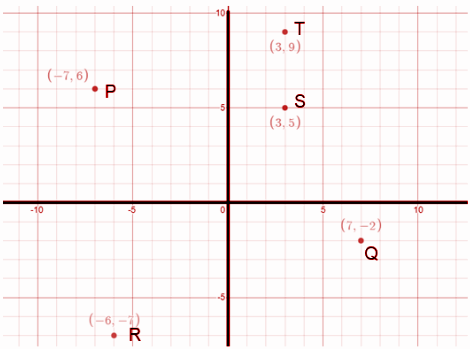P(-7, 6) lies in the second quadrant

Q(7, -2) lies in the first quadrant

R(-6, -7) lies in the third quadrant

S(3, 5) lies in the first quadrant

T(3, 9) lies in the first quadrant

Question 2: Write down the abscissa and ordinate of the following from the figure.

(i) P

(ii) Q

(iii) R

(iv) S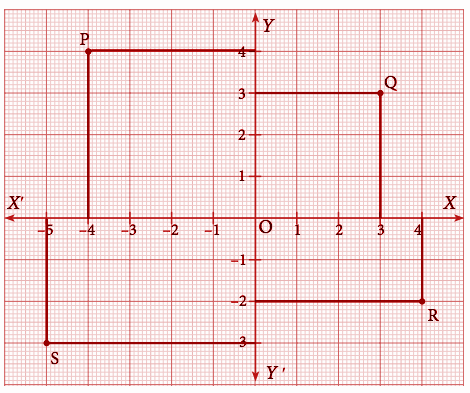Solution:

P (-4, 4)

Q (3, 3)

R (4, -2) and

S (-5, -3).

Question 3: Plot the following points in the coordinate plane and join them. What is your conclusion about the resulting figure?

(i) (–5,3) (–1,3) (0,3) (5,3)

(ii) (0,–4) (0,–2) (0,4) (0,5)

Solution:

(i)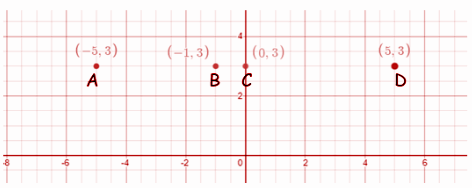The points are collinear. If the points are joined, a straight line is obtained which is parallel to the x-axis.

(ii)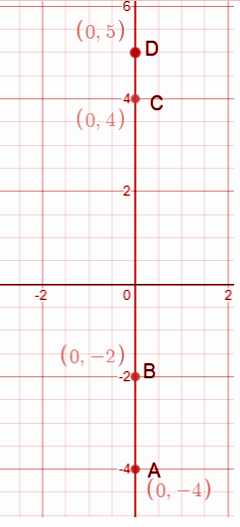A straight line lying on the y-axis is obtained.

Question 4: Plot the following points in the coordinate plane. Join them in order. What type of geometrical shape is formed?

(i) (0,0) (–4,0) (–4,–4) (0,–4)

(ii) (–3,3) (2,3) (–6,–1) (5,–1)

Solution:

(i)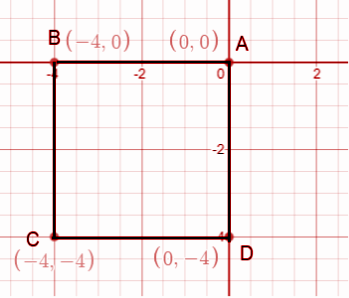A square is obtained when the points are joined.

(ii)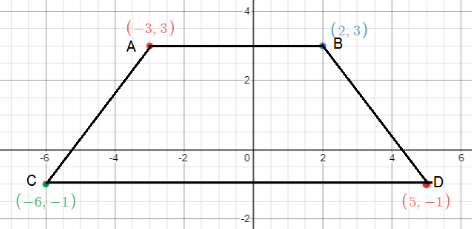A trapezium is obtained when the points are joined.

### Samacheer Kalvi 9th Maths Chapter 5: Geometry Book Exercise 5.2 Questions and Solutions

Question 1: Find the distance between the following pairs of points.

(i) (1, 2) and (4, 3)

(ii) (3,4) and (– 7, 2)

(iii) (a, b) and (c, b)

(iv) (3,– 9) and (–2, 3)

Solution:

(i) (1, 2) and (4, 3)

d = √(x2 – x1)2 + (y2 – y1)2

d = √(4 – 1)2 + (3 – 2)2

d = √(3)2 + (1)2

d = √9 + 1

d = √10 units

(ii) (3, 4) and (– 7, 2)

d = √(x2 – x1)2 + (y2 – y1)2

d = √(-7 – 3)2 + (2 – 4)2

d = √(-10)2 + (- 2)2

d = √100 + 4

d = √104

d = 2√26 units

(iii) (a, b) and (c, b)

d = √(c – a)2 + (b – b)2

d = √(c – a)2 + 0

d = √(c – a)2

d = (c – a) units

(iv) (3,– 9) and (–2, 3)

d = √(-2 – 3)2 + (3 + 9)2

d = √(-5)2 + (12)2

d = √(25) + (144)

d = √169

d = 13 units

Question 2: Determine whether the given set of points in each case are collinear or not.

(i) (7, –2), (5, 1), (3, 4)

(ii) (a, –2), (a, 3), (a, 0)

Solution:

(i) A (7, –2), B (5, 1), C (3, 4)

By distance formula,

AB = √(5 – 7)2 + (1 + 2)2 = √4 + 9 = √13

BC = √(3 – 5)2 + (4 – 1)2 = √4 + 9 = √13

CA = √(7 – 3)2 + (-2 – 4)2 = √16 + 36 = √52 = 2√13

AB + BC = CA

1√13 + 1√13 = 2√13

Hence, the points are collinear.

(ii) A (a, –2), B (a, 3), C (a, 0)

By distance formula,

AB = √(a – a)2 + (3 + 2)2 = √52 = √25 = 5

BC = √(a – a)2 + (0 – 3)2 = √0 + 9 = √9 = 3

CA = √(a – a)2 + (-2 – 0)2 = √0 + 4 = √4 = 2

AB = BC + CA

1√13 + 1√13 = 2√13

Hence, the points are collinear.

Question 3: Show that the following points are taken in order to form an isosceles triangle.

(i) A (5,4), B (2,0), C (–2,3)

(ii) A (6,–4), B (–2, –4), C (2,10)

Solution:

(i) A (5, 4), B (2, 0), C (–2, 3)

AB = √(2 – 5)2 + (0 – 4)2 = √9 + 16 = √25 = 5

BC = √(-2 -2)2 + (3 – 0)2 = √16 + 9 = √25 = 5

CA = √(5 + 2)2 + (4 – 3)2 = √49 + 1 = √50 = 5√2

AB + BC > CA

AB = BC

Hence, it is an isosceles triangle.

(ii) A (6,–4), B (–2, –4), C (2,10)

AB = √(2 – 6)2 + (-4 + 4)2 = √64 + 0 = √64 = 8

BC = √(2 + 2)2 + (10 + 4)2 = √16 + 196 = √212 = 2√53

CA = √(6 – 2)2 + (-4 – 10)2 = √16 + 196 = √212 = 2√53

BC + AB > CA

CA = BC

Hence, it is an isosceles triangle.

Question 4: Show that the following points are taken in order to form an equilateral triangle in each case.

(i) A (2, 2), B (–2, –2), C (-2√3, 2√3)

(ii) A (√3, 2), B (0,1), C (0,3)

Solution:

(i) A (2, 2), B (–2, –2), C (-2√3, 2√3)

AB = √(-2 – 2)2 + (-2 – 2)2 = √16 + 16 = √32 = 4√2

BC = √(-2√3 + 2)2 + (2√3 + 2)2 = √16 + 16 = √32 = 4√2

CA = √(2 + 2√3)2 + (2 – 2√3)2 = √16 + 16 = √32 = 4√2

AB = BC = CA = 4√2

CA = BC

All three sides are equal.

Hence, it is an equilateral triangle.

(ii) A (√3, 2), B (0,1), C (0,3)

AB = √(-2 – 2)2 + (-2 – 2)2 = √16 + 16 = √32 = 4√2

BC = √(-2√3 + 2)2 + (2√3 + 2)2 = √16 + 16 = √32 = 4√2

CA = √(2 + 2√3)2 + (2 – 2√3)2 = √16 + 16 = √32 = 4√2

AB = BC = CA = 4√2

CA = BC

All three sides are equal.

Hence, it is an equilateral triangle.

Question 5: Show that the following points taken in order form the vertices of a parallelogram.

(i) A(–3, 1), B(–6, –7), C (3, –9), D(6, –1)

(ii) A (–7, –3), B(5,10), C(15,8), D(3, –5)

Solution:

(i) A(–3, 1), B(–6, –7), C (3, –9), D(6, –1)

AB = √(-6 + 3)2 + (-7 – 1)2 = √9 + 64 = √73

BC = √(3 + 6)2 + (-9 + 7)2 = √81 + 4 = √85

CD = √(6 – 3)2 + (-1 + 9)2 = √9 + 64 = √73

DA = √(-3 – 6)2 + (1 + 1)2 = √81 + 4 = √85

AB = CD = √73

DA = BC = √85

The opposite sides are equal.

Hence, it is a parallelogram.

(ii) A (–7, –3), B(5,10), C(15,8), D(3, –5)

AB = √(5 + 7)2 + (10 + 3)2 = √144 + 169 = √313

BC = √(15 – 5)2 + (8 – 10)2 = √100 + 4 = √104

CD = √(3 – 15)2 + (-5 – 8)2 = √144 + 169 = √313

DA = √(-7 – 3)2 + (-3 + 5)2 = √100 + 4 = √104

AB = CD = √313

DA = BC = √104

The opposite sides are equal.

Hence, it is a parallelogram.

Question 6: Verify that the following points taken in order form the vertices of a rhombus.

(i) A (3,–2), B (7,6), C (–1,2), D (–5, –6)

(ii) A (1,1), B (2,1), C (2,2), D (1,2)

Solution:

(i) A (3,–2), B (7,6), C (–1,2), D (–5, –6)

AB = √(7 – 3)2 + (6 + 2)2 = √16 + 64 = √80 = 4√5

BC = √(-1 – 7)2 + (2 – 6)2 = √64 + 16 = √80 = 4√5

CD = √(-5 + 1)2 + (-6 – 2)2 = √16 + 64 = √80 = 4√5

DA = √(3 + 5)2 + (-2 + 6)2 = √64 + 16 = √80 = 4√5

All the four sides of a quadrilateral are equal.

Hence, it is a rhombus.

(ii) A (1,1), B (2,1), C (2,2), D (1,2)

AB = √(2 – 1)2 + (1 – 1)2 = √1 + 0 = √1 = 1

BC = √(2 – 2)2 + (2 – 1)2 = √0 + 1 = √1 = 1

CD = √(1 – 2)2 + (2 – 2)2 = √1 + 0 = √1 = 1

DA = √(1 – 1)2 + (1 – 2)2 = √0 + 1 = √1 = 1

All the four sides of a quadrilateral are equal.

Hence, it is a rhombus.

Question 7: A (–1, 1), B (1, 3) and C (3, a) are points and if AB = BC, then find ‘a’.

Solution:

A (–1, 1), B (1, 3) and C (3, a)

AB = BC

AB = √(1 + 1)2 + (3 – 1)2 = √4 + 4 = √8

BC = √(3 – 1)2 + (a – 3)2 = √4 + a2 – 6a + 9 = √a2 – 6a + 13

AB = BC

√8 = √a2 – 6a + 13

By squaring on both sides,

8 = a2 – 6a + 13

a2 – 6a + 5 = 0

(a – 1) (a – 5) = 0

a = 1, 5

Question 8: The abscissa of a point A is equal to its ordinate, and its distance from the point B (1, 3) is 10 units, What are the coordinates of A?

Solution:

BA = √(x2 – 1)2 + (y2 – 3)2 = 10

√(x22 – 2x2 + 1 + y22 – 6y + 9) = 10

Since x2 = y2

√(x22 – 2x2 + 1 + x22 – 6x2 + 9) = 10

√2x22 – 8x2 + 10 = 100

On squaring of both sides,

2x22 – 8x2 + 10 = 10

2x22 – 8x2 – 90 = 0

x22 – 4x2 – 45 = 0

(x2 + 5) (x2 – 0) = 0

The coordinates of A are (-5 , -5) and (9, 9).

Question 9: The point (x, y) is equidistant from the points (3, 4) and (–5, 6). Find a relation between x and y.

Solution:

P (x, y) is equidistant from the points A (3, 4) and B (-5, 6).

PA = PB

√(x – 3)2 + (y – 4)2 = √(x – (-5))2 + (y – 6)2

x2 – 6x + 9 + y2 – 8y + 16 = x2 + 10x + 25 + y2 – 12y + 36

16x – 4y + 36 = 0

4x – y + 9 = 0

y = 4x + 9

Question 10: Let A (2, 3) and B (2, –4) be two points. If P lies on the x-axis, such that AP = (3 / 7) AB, find the coordinates of P.

Solution:

A (2, 3), B (2, -4), P (x, y) lies on the x-axis, P (x, 0).

AP = (3 / 7) AB

√(x – 2)2 + (0 – 3)2 = (3 / 7) √(x – 2)2 + (0 – 3)2

√x2 – 4x + 4 + 9 = (3 / 7) √(-7)2

7 (√x2 – 4x + 13) = 3 * 7

√x2 – 4x + 13 = 3

By squaring on both sides,

x2 – 4x + 13 = 9

x2 – 4x + 13 – 9 = 0

x2 – 4x + 4 = 0

(x – 2)2 = 0

x = 2, 2

The coordinates of P (x, 0) is P (2, 0).

Question 11: Show that the point (11, 2) is the centre of the circle passing through the points (1, 2), (3, –4) and (5, –6).

Solution: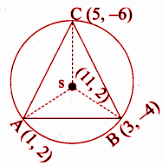To show that SA = SB = SC

d = √(x2 – x1)2 + (y2 – y1)2

SA = √(1 – 11)2 + (2 – 2)2 = √(10)2 + (0)2 = √100 = 10 units

SB = √(3 – 11)2 + (-4 – 2)2 = √(-8)2 + (-6)2 = √100 = 10 units

SC = √(5 – 11)2 + (-6 – 2)2 = √(-6)2 + (-8)2 = √100 = 10 units

SA = SB = SC = 10 units

Hence, S is the centre of the circle, passing through A, B and C.

Question 12: The radius of a circle with centre at origin is 30 units. Write the coordinates of the points where the circle intersects the axes. Find the distance between any two such points.

Solution: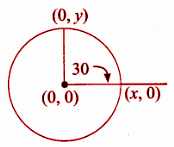Equation of the circle = √x2 + y2 = 30

Radius = SA = 30 units

√(x – 0)2 + (0 – 0)2 = 30

√x2 + 02 = 30

On squaring both sides,

x2 + 02 = 900

x2 = 900

x = ± 30

√(0 – 0)2 + (y – 0)2 = 30

√y2 + 02 = 30

On squaring both sides,

y2 + 02 = 900

y2 = 900

y = ± 30

The distance between the points (30 ,0) and (0, 30) is

√(0 – 30)2 + (30 – 0)2 = √(-30)2 + (30)2 = √900 + 900 = √1800 = 30√2

### Samacheer Kalvi 9th Maths Chapter 5: Geometry Book Exercise 5.3 Questions and Solutions

Question 1: Find the midpoint of the line segment joining the points (−2, 3) and (−6, −5)

Solution:

(x1, y1) => (-2, 3)

(x2 , y2) => (-6, -5)

Midpoint = (-2 – 6) / 2, (3 + (-5)) / 2

= -8 / 2, -2 / 2

= (-4, -1)

Question 2: The centre of a circle is (−4, 2). If one end of the diameter of the circle is (−3, 7), then find the other end.

Solution:

The midpoint of the diameter = Center of the circle

Let the other endpoint be (a, b).

Midpoint of (-3, 7) and (a, b) is (-4, 2).

(-3 + a) / 2, (7 + b) / 2 = (-4, 2)

By equating the x and y coordinates, we get

(-3 + a) / 2 = -4

-3 + a = -8

a = -8 + 3

a = -5

(7 + b) / 2 = 2

7 + b = 4

b = 4 – 7

b = -3

Question 3: If the midpoint (x,y) of the line joining (3,4) and (p,7) lies on 2x + 2y + 1= 0, then what will be the value of p?

Solution:

Midpoint of the line segment joining the points (3, 4) and (p, 7)

(3 + p) / 2 , (7 + 4) / 2 = (x, y)

(3 + p) / 2 , 11 / 2 = (x, y)

x = (3 + p) / 2 and y = 11 / 2

Since the midpoint lies on the line 2x + 2y + 1 = 0

2 (3 + p) / 2 + 2 (11 / 2) + 1 = 0

3 + p + 11 + 1 = 0

p + 15 = 0

p = -15

Question 4: The midpoint of the sides of a triangle are (2, 4), (−2, 3) and (5, 2). Find the coordinates of the vertices of the triangle.

Solution:

Let (x1, y1) => (2, 4)

(x2, y2) => (-2, 3) and (x3, y3) => (5, 2)

By using the formula given above,

A (x1 + x3 – x2, y1 + y3 – y2) => [(2 + 5 + 2), (4 + 2 -3)] = (9, 3)

B (x1 + x2 – x3, y1 + y2 – y,) => [(2 -2 -5), (4 + 3 -2)] = (-5, 5)

C (x2 + x3 – x1, y2 + y3 – y1) => [(-2 + 5 -2), (3 + 2 -4)] = (1, 1)

Hence, the required vertices are (9, 3) (-5, 5) and (1, 1).

Question 5: O (0,0) is the centre of a circle whose one chord is AB, where the points A and B are (8, 6) and (10, 0), respectively. OD is the perpendicular from the centre to the chord AB. Find the coordinates of the mid-point of OD.

Solution: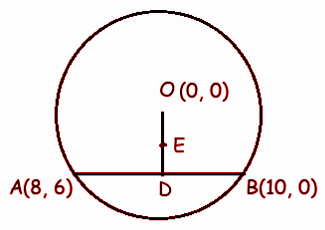The midpoint of the chord AB = D

(x1 + x2) / 2, (y1 + y2) / 2

= (8 + 10 ) / 2, (6 + 0) / 2

= 18 / 2, 6 / 2

= (9, 3)

To find the midpoint of OD, that is E

E = (0 + 9) / 2, (0 + 3) / 2

= (9 / 2, 3 / 2)

Question 6: The points A (−5, 4), B (-1, -2) and C(5, 2) are the vertices of an isosceles right-angled triangle where the right angle is at B. Find the coordinates of D so that ABCD is a

square.

Solution: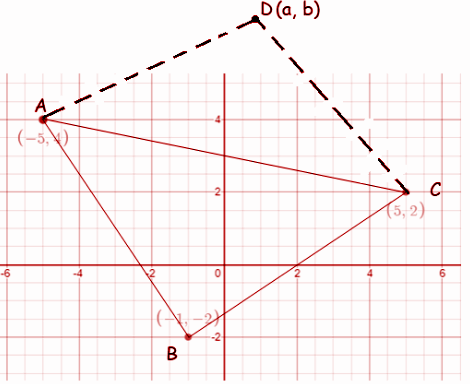It forms a square.

The midpoint of the diagonal AC and BD are equal.

The midpoint of AC:

A (-5, 4) C (5, 2)

= (-5 + 5) / 2, (4 + 2) / 2

= 0 / 2, 6 / 2

= (0, 3)

The midpoint of BD:

B (-1, -2) D (a, b)

= (-1 + a) / 2, (-2 + b) / 2

By equating the x and y coordinates, we get

(-1 + a) / 2 = 0

-1 + a = 0

a = 1

(-2 + b) / 2 = 3

-2 + b = 6

b = 6 + 2

b = 8

Hence, the required vertex is (1, 8).

Question 7: The points A (−3, 6), B (0, 7) and C (1, 9) are the mid-points of the sides DE, EF and FD of a triangle DEF. Show that the quadrilateral ABCD is a parallelogram.

Solution: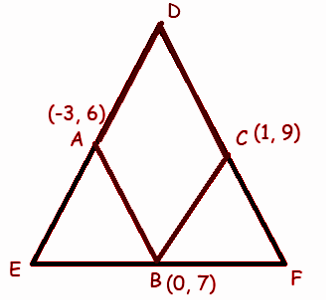In order to prove that ABCD is a parallelogram, point D has to be found.

= D (-3 + 1 – 0, 6 + 9 – 7)

= D (-2, 8)

In ABCD is a parallelogram, then the midpoint of diagonals AC and BD will be equal.

Midpoint of AC = (-3 + 1) / 2, (6 + 9) / 2

= -2 / 2, 15 / 2

= (-1, 15 / 2)

Midpoint of BD

B (0, 7) and D (-2, 8)

= (0 – 2) / 2, (7 + 8) / 2

= -2/2, 15/2

= (-1, 15 / 2)

Hence, ABCD is a parallelogram.

Question 8: A (−3, 2), B (3, 2) and C (-3, -2) are the vertices of the right triangle, right-angled at A. Show that the midpoint of the hypotenuse is equidistant from the vertices.

Solution: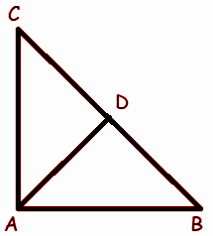Midpoint of BC = (3 + (-3)) / 2, (2 + (-2)) / 2 = D (0, 0)

A (-3, 2) D (0, 0)

= √(x2 – x1)2 + (y2 – y1)2

= √(-3 -0)2 + (2 – 0)2

= √9 + 4

= √13

C (-3, -2) D (0, 0)

= √(x2 – x1)2 + (y2 – y1)2

= √(-3 -0)2 + (-2 -0)2

= √9 + 4

= √13

B (3, 2) D (0, 0)

= √(x2 – x1)2 + (y2 – y1)2

= √(0 – 3)2 + (0 – 2)2

= √9 + 4

= √13

Hence the midpoint of the hypotenuse is equidistant from the vertices.